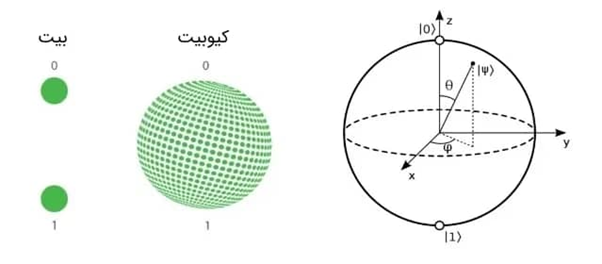Select Page

#### Law Physics Quantum (LPQ)

Legal and contractual matters of using this software:

Dear user, welcome to your favorite website. Thank you for choosing this website as your personal advisor in science (physics, mechanics, mathematics).

In this scientific software, if you want to move a moving particle on a path with the speed of light and this type of movement has permanent motion relative to a fixed point (origin of coordinates), you can use this software for free (subject to Use the following criteria on your personal computer. This software is the basic software for preparing your personal software.

Bloch Sphere

For an intuitive understanding of the state of a qubit, it can be convenient to represent it on a Bloch sphere. In fact, a qubit can occupy any place on the surface of the Bloch sphere at the same time. While a classical bit has only two values ​​0 and 1 on the poles of the Bloch sphere.

By defining the coefficients α and β in the following form, the state of a qubit can be displayed on the Bloch sphere. We remind you that before measuring the system, the location of the qubit can be anywhere on the surface of the Bloch sphere. In fact, by measuring, i.e. determining the coefficients, the exact location and position of the qubit can be determined.

α=cos(θ2) α=cos(θ2)

β=eiϕsin(θ2) β=eiϕsin(θ2)Now it seems that the answer to the question mentioned in the introduction of the text can be given according to the above explanations and the green sphere in the above figure. Yes, you guessed it, parallel processing and more speed!

The laws of quantum physics

(Laws of subatomic motions)

Review:

The common chapter of the three classical theories, quantum mechanics and general relativity can be proved in three chapters separately, and then the relationship between the three chapters can be presented.

Proving the classical theory: Proving the trigonometry of a point in space or proving the hexadecimal relations assuming (x,y,z)

Proof of the relevant formulas in terms of speed.

A: Constant speed

B: variable speed

Quantum theory: integration of three classical theories, general relativity and stable dynamics (the fifth dimension)

Introduction:

A theory that can explain all laws and phenomena in the world can include quantum gravity.

The integration of quantum mechanics and general relativity cannot be justified with today’s science and knowledge, but in order to fully explain quantum gravity, it is necessary to present a new theory that is the intersection of 3 classical theories, quantum mechanics and general relativity.

Proof of classical theory:

Proof of six angles in space in terms of (x,y,z) relative to the point (o). More explanations in this field are proven in the trigonometry section of www.p3m.ir website.